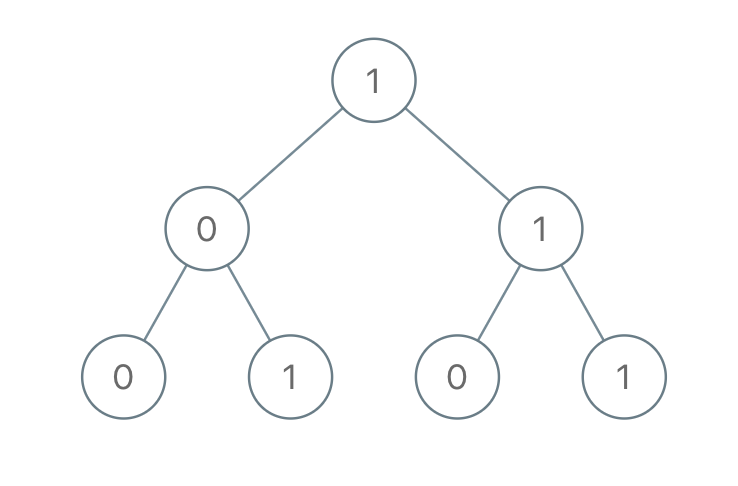# LeetCode Weekly Contest 131

https://leetcode.com/contest/weekly-contest-131

## Remove Outermost Parentheses

A valid parentheses string is either empty (""), "(" + A + ")", or A + B, whereA and Bare valid parentheses strings, and + represents string concatenation. For example, "", "()", "(())()", and "(()(()))" are all valid parentheses strings.

A valid parentheses string S is primitive if it is nonempty, and there does not exist a way to split it into S = A+B, with A and B nonempty valid parentheses strings.

Given a valid parentheses string S, consider its primitive decomposition: S = P_1 + P_2 + ... + P_k, where P_i are primitive valid parentheses strings.

Return S after removing the outermost parentheses of every primitive string in the primitive decomposition of S.

Example 1:

Example 2:

Example 3:

Note:

1. S.length <= 10000
2. S[i] is "(" or ")"
3. S is a valid parentheses string

## Sum of Root To Leaf Binary Numbers

Given a binary tree, each node has value 0 or 1. Each root-to-leaf path represents a binary number starting with the most significant bit. For example, if the path is 0 -> 1 -> 1 -> 0 -> 1, then this could represent 01101 in binary, which is 13.

For all leaves in the tree, consider the numbers represented by the path from the root to that leaf.

Return the sum of these numbers.

Example 1:Note:

1. The number of nodes in the tree is between 1 and 1000.
2. node.val is 0 or 1.
3. The answer will not exceed 2^31 - 1.

DFS找到每个到叶结点的路径对应的二进制数，遍历到叶结点时直接把路径代表的数字加到最终结果。

## Camelcase Matching

A query word matches a given pattern if we can insert lowercase letters to the pattern word so that it equals the query. (We may insert each character at any position, and may insert 0 characters.)

Given a list of queries, and a pattern, return an answer list of booleans, where answer[i] is true if and only if queries[i] matches the pattern.

Example 1:

Example 2:

Example 3:

Note:

1. 1 <= queries.length <= 100
2. 1 <= queries[i].length <= 100
3. 1 <= pattern.length <= 100
4. All strings consists only of lower and upper case English letters.

## Video Stitching

You are given a series of video clips from a sporting event that lasted T seconds. These video clips can be overlapping with each other and have varied lengths.

Each video clip clips[i] is an interval: it starts at time clips[i] and ends at time clips[i]. We can cut these clips into segments freely: for example, a clip [0, 7] can be cut into segments [0, 1] + [1, 3] + [3, 7].

Return the minimum number of clips needed so that we can cut the clips into segments that cover the entire sporting event ([0, T]). If the task is impossible, return -1.

Example 1:

Example 2:

Example 3:

Example 4:

Note:

1. 1 <= clips.length <= 100
2. 0 <= clips[i], clips[i] <= 100
3. 0 <= T <= 100

dp[i]表示保证覆盖到第i秒时最少需要多少clip，如果有一个区间为[x,y]的clip，且x<=i，那么dp[i]+1可以作为dp[y]的一个备选。先把区间按照x排序，然后计算每个[x,y]可以续接哪些i，然后更新dp[y]以及iy之间所有dp不超过dp[y]，最后检查dp[T]是否还是未被更新的默认值，如果是则返回-1，否则直接返回dp[y]

x1<x2<y2<y1时，可以把[x2,y2]合并到[x1,y1]，只考虑[x1,y1]，那么在排序时可以直接以(x,-y)排序，把排序后y值小于前面的元素的去掉，可以减少部分工作量。